## Lesson 6 – Focal Length and Applications of Lenses

Posted on: Saturday, March 5th, 2022  In: Learning Optics with Austin

Focal length is important not just in terms of positive and negative. For convex lenses, different focal lengths can have drastically different images. If an object (u) is at a distance greater than two times its focal length (2f), then its image focal length (v) is between f and 2f (written as f < v < 2f). The image will appear upside down and smaller than the object. This is the case for cameras, of which are a primary application of this property.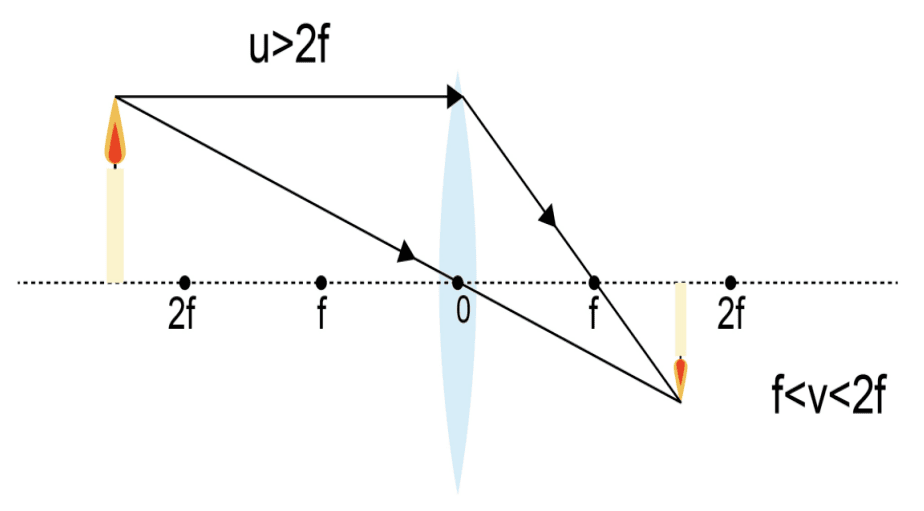u > 2f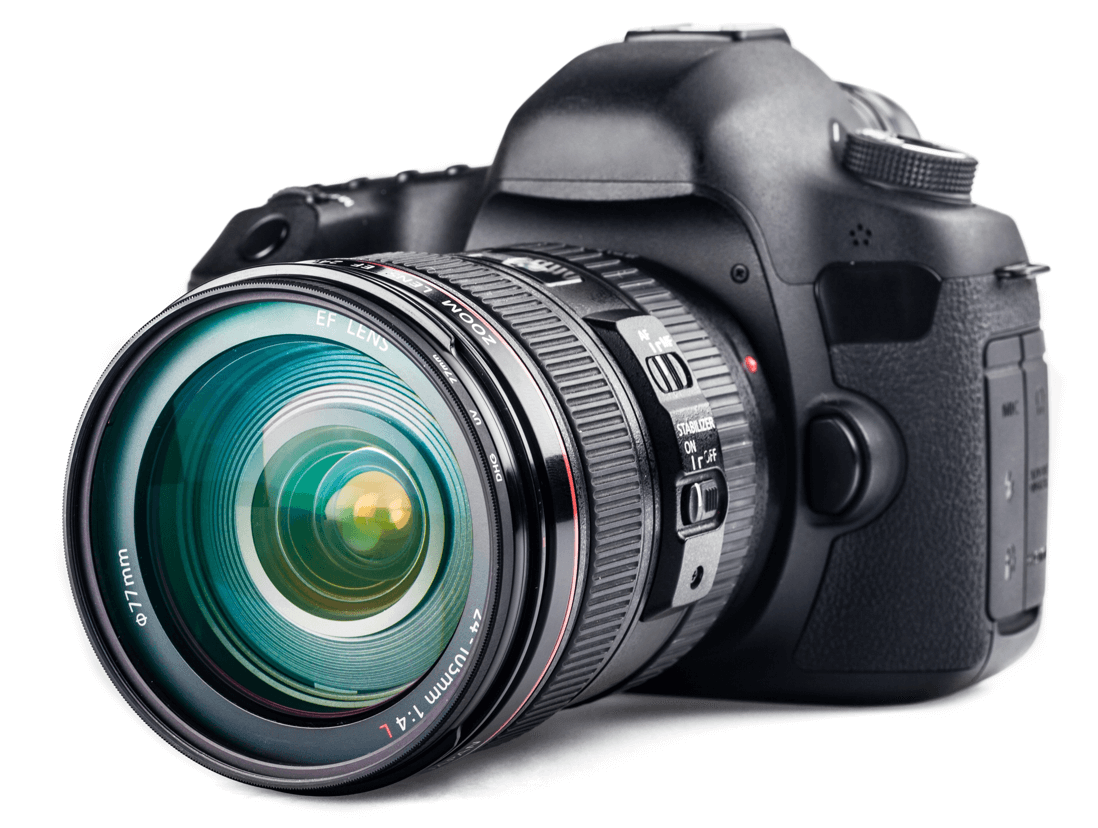Camera

If however, the object is at exactly the distance of two times the focal length (u = 2f), then the image focal length is exactly twice the focal length (v = 2f). The image is still upside down, but is the same size as the object. Scanners and printers are primary examples of this application.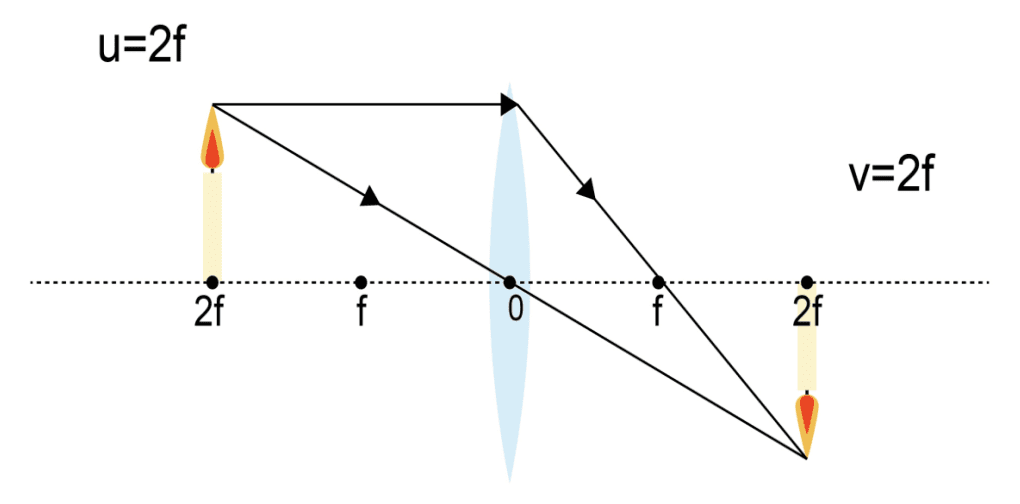u = 2fScanner

When the object is at a length less than 2 focal lengths but greater than a focal length (f < u < 2f), the image focal length is greater than 2 focal lengths (v > 2f). While the image is upside down, it is larger than the original object. This property is utilized by projectors in displaying an image much larger than the original object.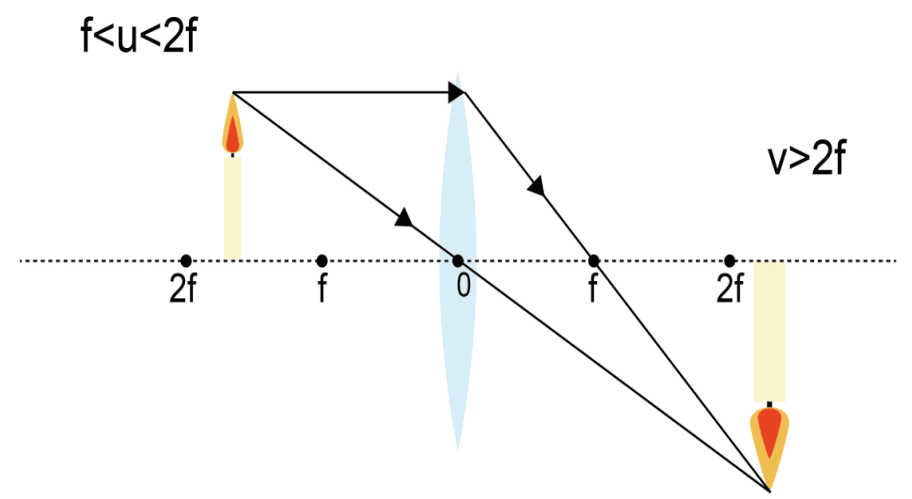f < u < 2f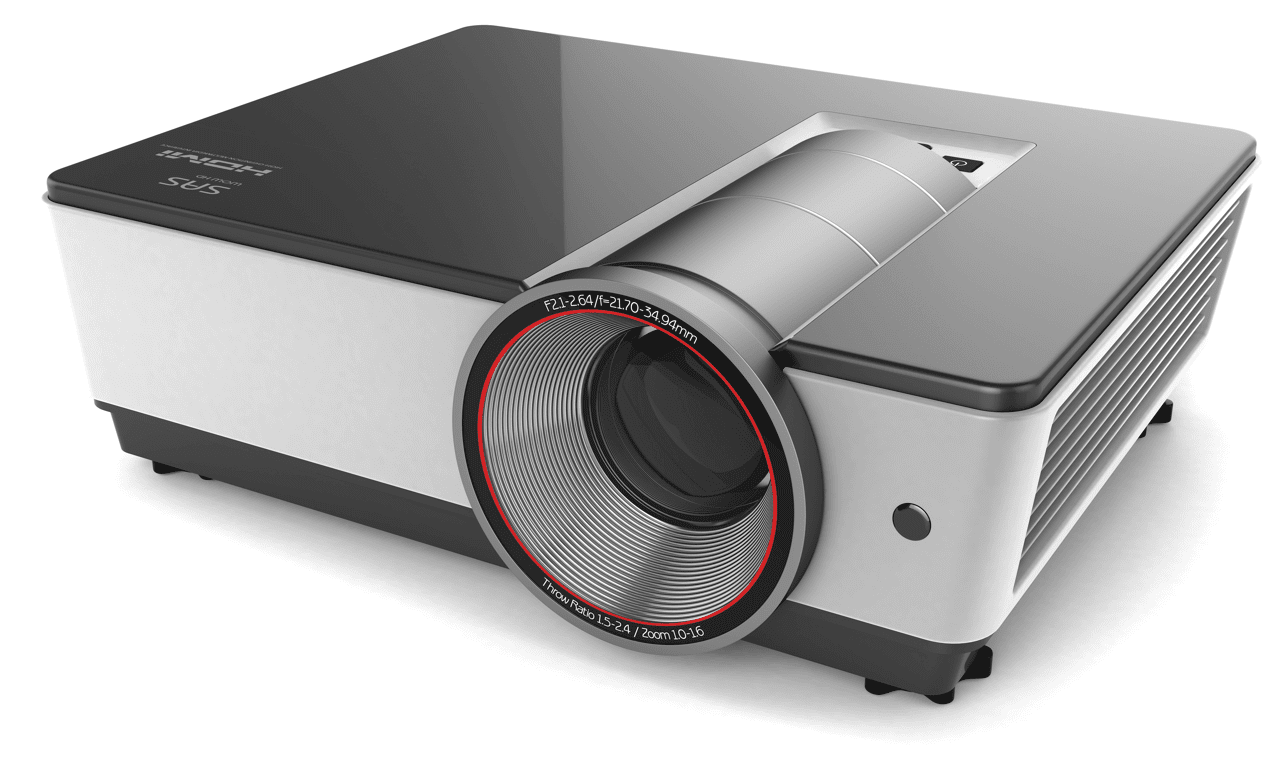Projector

If an object is less than a focal length away from the lens (u < f), no real image exists. However, a virtual image does exist and is larger than the actual object. An example of this phenomenon is a magnifying glass which enlarges the image to look bigger than the object itself.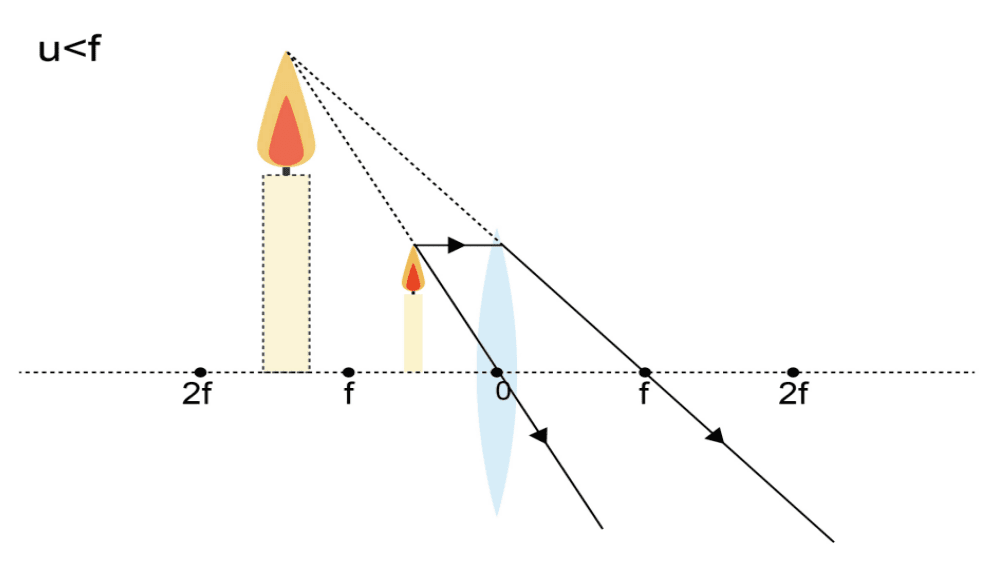u < fMagnifying Glass

There is also the case where the object is exactly a focal length away from the lens (u = f). As light runs parallel through the lens at this distance, no image or virtual image exists.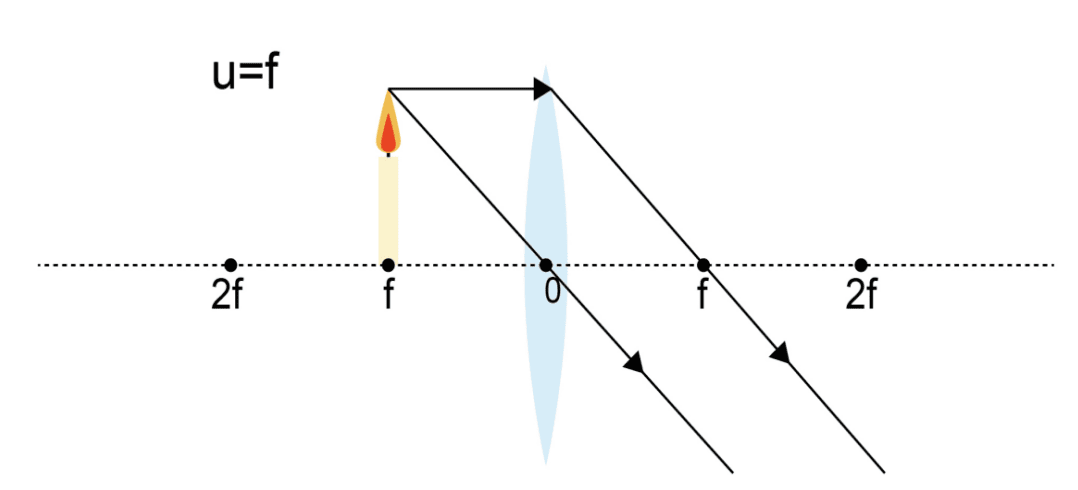u = f

Every case above has been under the assumption that a convex lens is used. For concave lenses, no matter where you place the object, the image will always be smaller than the actual object. For people with nearsightedness, their glasses are concave lenses. The image through their glasses will always be smaller than the object itself.Telescope

Optical instruments such as telescopes are not composed of a single lens. Instead, multiple lenses are assembled together to produce the desired optical effect. In the next post, we will be discussing an example of an assembled system and specifications when designing lenses.

Next: Lesson 7 – The Eyepiece and Lens Requirements